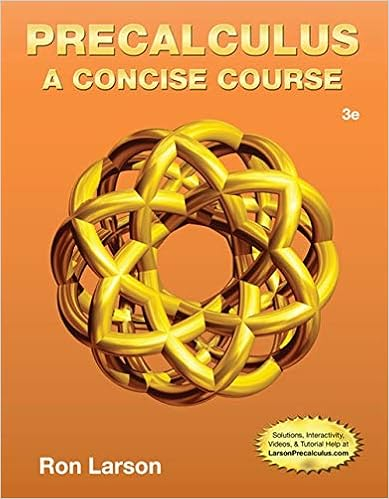# 3 230217 math 040 re entry mathematics franklin

• Homework Help
• 6
• 100% (1) 1 out of 1 people found this document helpful

This preview shows page 3 - 6 out of 6 pages.

##### We have textbook solutions for you!
The document you are viewing contains questions related to this textbook.The document you are viewing contains questions related to this textbook.
Chapter 2 / Exercise 7
Precalculus: A Concise Course
LarsonExpert Verified
3230217
##### We have textbook solutions for you!
The document you are viewing contains questions related to this textbook.The document you are viewing contains questions related to this textbook.
Chapter 2 / Exercise 7
Precalculus: A Concise Course
LarsonExpert Verified
Math 040 Re-Entry Mathematics Franklin University16.Complete each table to make solutions to the given equation.
17. Graph each equation by first completing the table of solutions.
Month, xTemperature (°C), y1−72−3314651261771881891410611−112−7xyxy
Math 040 Re-Entry Mathematics Franklin University18. Graph the equation.
19. Given the equation 2x+ y= 4,a.Complete the ordered pairs (0, ) and (, 0).b.Graph the ordered pairs from part (a) and draw the line through the points.c.Which point is the x-intercept? Which point is the y-intercept?5230217
Math 040 Re-Entry Mathematics Franklin University20.Given the equation x− 4y= 8, a.Complete the ordered pairs (0, ) and (, 0).b.Graph the ordered pairs from part (a) and draw the line through the points.c.Which point is the x-intercept? Which point is the y-intercept?6230217
•••